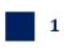Smartick is an advanced online program that teaches kids math and coding in only 15 min. a day

Jun04

# Representing Polynomials with Algebra Tiles

Today we’re going to take a look at a very useful system of representing and working with monomials and polynomials: algebra tiles. They’re very intuitive and easy to use, and we’re currently incorporating them into our platform so that our students have this resource available when working with polynomials in their daily Smartick sessions.

If you’re not sure what a polynomial is, take a look at our previous entry about its properties before you continue.

#### Representing polynomials with algebra tiles

Algebra tiles are tiles of different colors and sizes. Each size corresponds to a degree of the different monomials of the polynomial. An example will make it easier to understand:

• This tile represents a unit. It corresponds to monomials with a degree of 0.• This represents ‘x’. It corresponds to monomials with a degree of 1.• Finally, this represents x2. It corresponds to monomials with a degree of 2.Therefore, if we want to represent the polynomial 2x+ 4x + 5 it’s as easy as arranging the corresponding tiles:We’ve got 2 tiles of ‘x24 tiles of ‘x’, and 5 tiles that represent a unit.

Easy, right?

#### Adding polynomials with algebra tiles

Adding polynomials is just as simple as representing them. In this case we’ll use polynomials with all positive monomials. We’ll take the polynomial that we represented before, 2x+ 4x + 5, as the first summand. The second summand will be x+ 2x + 3. Let’s represent them both with the algebra tiles:Now we add the like tiles to find the result…The polynomial result of the addition is 3x+ 6x + 8.

It’s an easy and intuitive resource that helps us better understand polynomials and operations with them. You can make them yourself and practice with them anywhere you want!

If you want to know more about polynomials and other mathematical content, log in to Smartick and try it free.